### Home > CAAC > Chapter 1 > Lesson 1.1.3 > Problem1-25

1-25.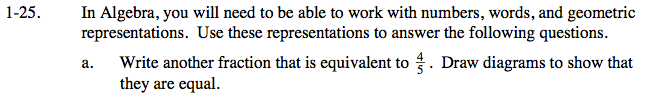Try multiplying by a fraction equal to 1.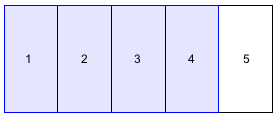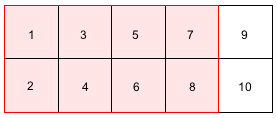$\frac{4}{5}\cdot \frac{2}{2}=\frac{8}{10}$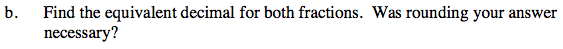$\frac{8}{10}=0.8$

Rounding was not necessary.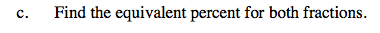Percent means 'per hundred' or 'out of a hundred'.

Try setting it up as a proportion to 100.

Multiply the top and bottom by 20 to make the denominatorequal to 100 and solve for x.

$\frac{4}{5}=\frac{x}{100}$

$\frac{4}{5}\cdot \frac{20}{20}=\frac{80}{100}$

x = 80 so,
x = 80%